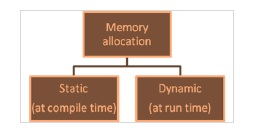# What is Realloc in C language?

CServer Side ProgrammingProgramming

The C library memory allocation function void *realloc(void *ptr, size_t size) attempts to resize the memory block pointed to by ptr that was previously allocated with a call to malloc or calloc.

## Memory allocation Functions

Memory can be allocated in two ways as explained below −Once memory is allocated at compile time, it cannot be changed during execution. There will be a problem of either insufficiency or else wastage of memory.

The solution is to create memory dynamically i.e. as per the requirement of the user during execution of program.

The standard library functions which are used for dynamic memory management are as follows −

• malloc ( )
• calloc ( )
• realloc ( )
• free ( )

## The realloc ( ) function

• It is used for reallocating already allocated memory.

• It can either decrease or increase the allocated memory.

• It returns a void pointer that points to the base address of reallocated memory.

The syntax for realloc() function is as follows −

Free void *realloc (pointer, newsize);

## Example

The following example shows the usage of realloc() function.

int *ptr;
ptr = (int * ) malloc (1000);// we can use calloc also
- - -
- - -
- - -
ptr = (int * ) realloc (ptr, 500);
- - -
- - -
ptr = (int * ) realloc (ptr, 1500);

## Example

Given below is the C program using realloc () function −

Live Demo

#include<stdio.h>
#include<stdlib.h>
int main(){
int *ptr, i, num;
printf("array size is 5\n");
ptr = (int*)calloc(5, sizeof(int));
if(ptr==NULL){
printf("Memory allocation failed");
exit(1); // exit the program
}
for(i = 0; i < 5; i++){
printf("enter number at %d: ", i);
scanf("%d", ptr+i);
}
printf("\nLet's increase the array size to 7\n ");
ptr = (int*)realloc(ptr, 7 * sizeof(int));
if(ptr==NULL){
printf("Memory allocation failed");
exit(1); // exit the program
}
printf("\n enter 2 more integers\n\n");
for(i = 5; i < 7; i++){
printf("Enter element number at %d: ", i);
scanf("%d", ptr+i);
}
printf("\n result array is: \n\n");
for(i = 0; i < 7; i++){
printf("%d ", *(ptr+i) );
}
return 0;
}

## Output

When the above program is executed, it produces the following result −

array size is 5
enter number at 0: 23
enter number at 1: 12
enter number at 2: 45
enter number at 3: 67
enter number at 4: 20
Let's increase the array size to 7
enter 2 more integers
Enter element number at 5: 90
Enter element number at 6: 60
result array is:
23 12 45 67 20 90 60